Volume Of Cone

Chapter 13 Class 9 Surface Areas and Volumes
Concept wise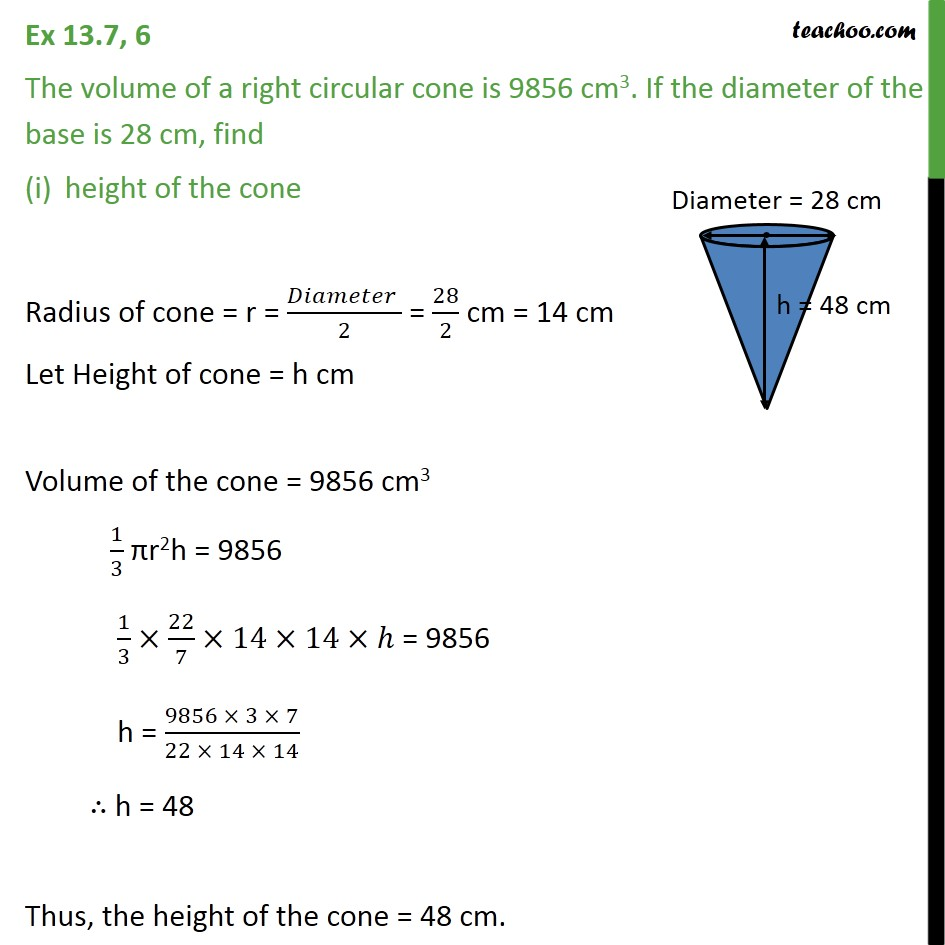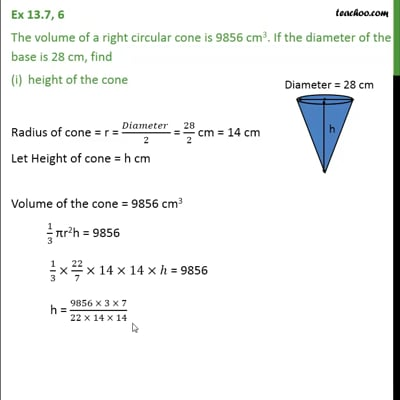This video is only available for Teachoo black users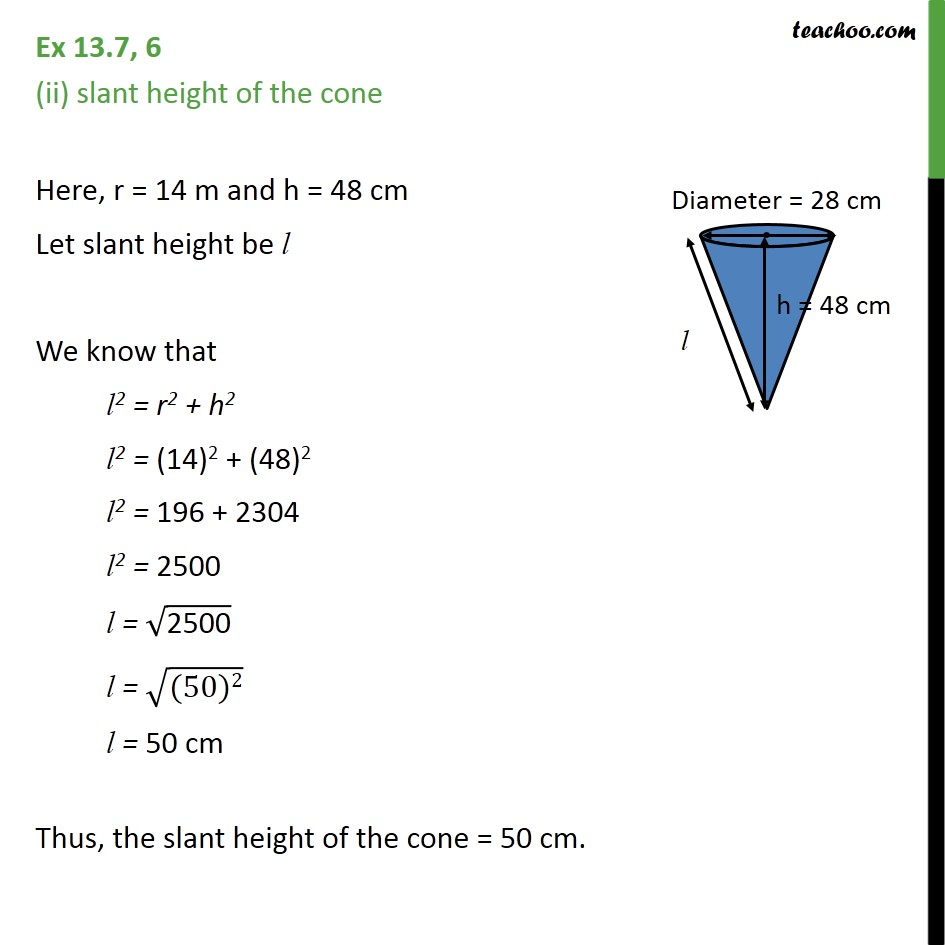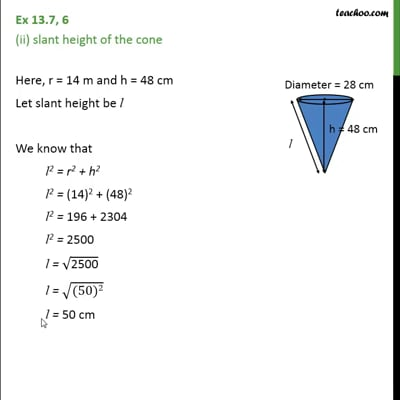This video is only available for Teachoo black users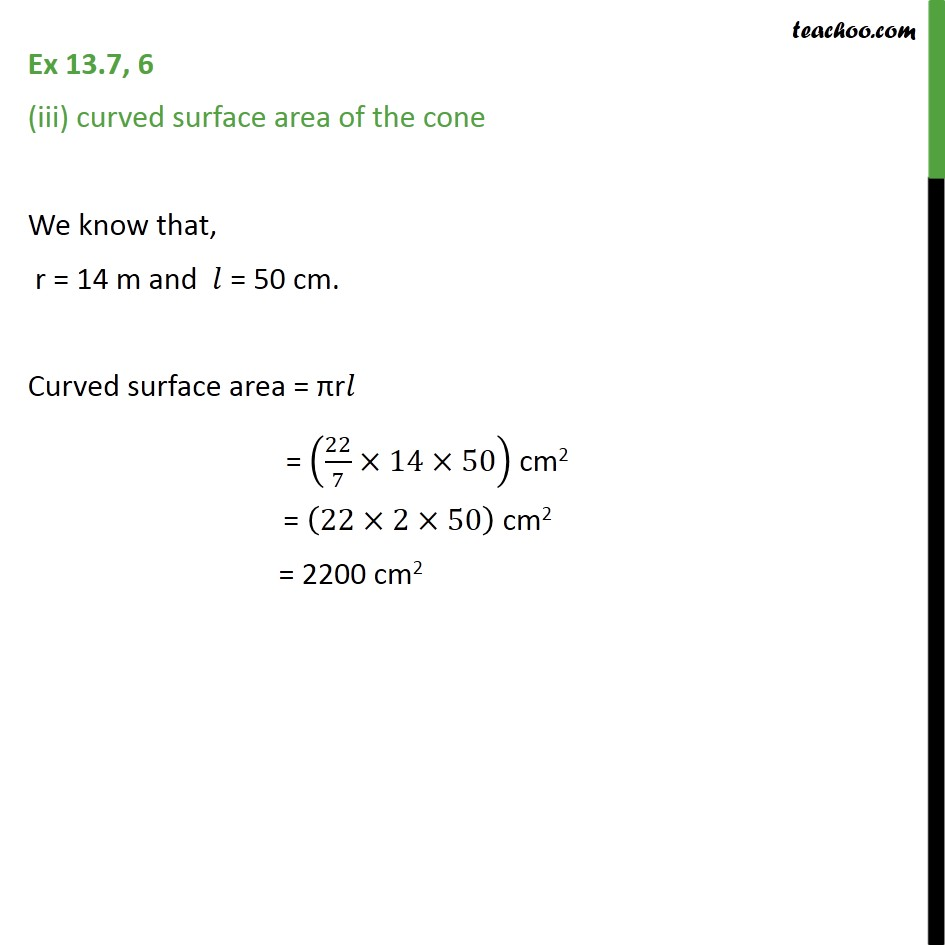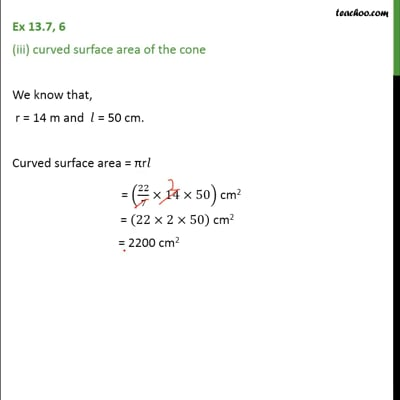This video is only available for Teachoo black users

Introducing your new favourite teacher - Teachoo Black, at only ₹83 per month

### Transcript

Ex 13.7, 6 The volume of a right circular cone is 9856 cm3. If the diameter of the base is 28 cm, find height of the cone Radius of cone = r = = cm = 14 cm Let Height of cone = h cm Volume of the cone = 9856 cm3 πr2h = 9856 = 9856 h = ∴ h = 48 Thus, the height of the cone = 48 cm. Ex 13.7, 6 (ii) slant height of the cone Here, r = 14 m and h = 48 cm Let slant height be l We know that l2 = r2 + h2 l2 = l2 = l2 = l = l = l = 50 cm Thus, the slant height of the cone = 50 cm. Ex 13.7, 6 (iii) curved surface area of the cone We know that, r = 14 m and = 50 cm. Curved surface area = πr = cm2 = cm2 = 2200 cm2